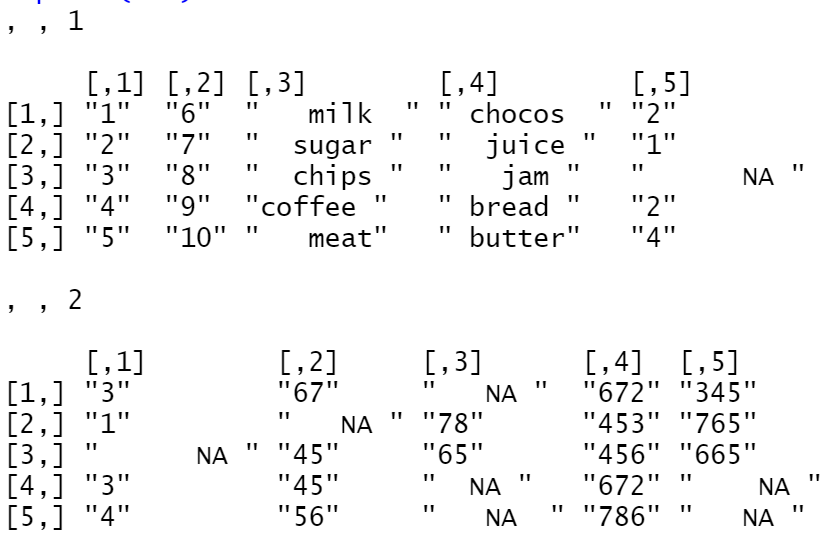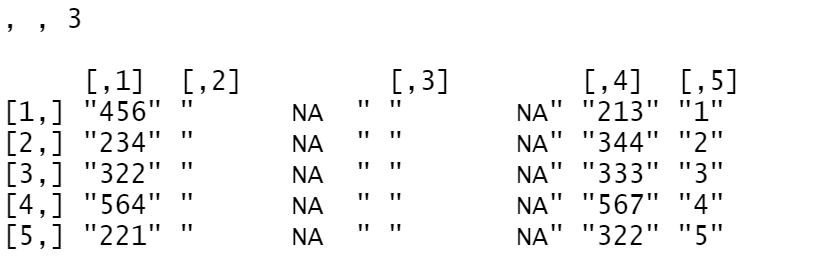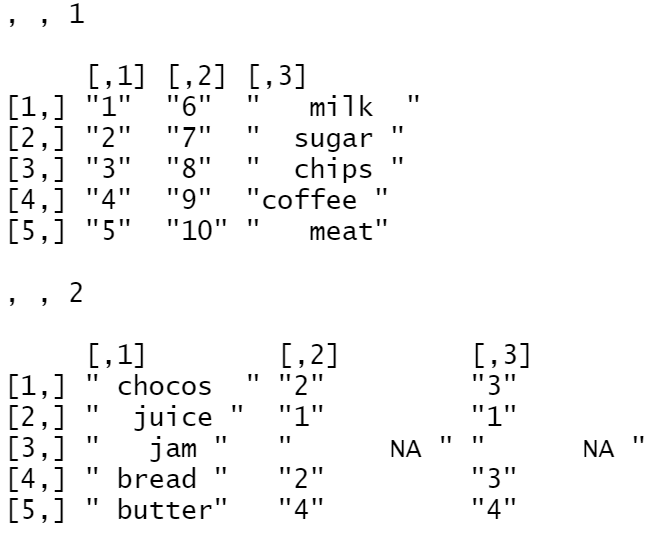# How to convert CSV into array in R?

• Last Updated : 02 Jul, 2021

In this article, we are going to see how to convert CSV into an array in R Programming Language. If we load this file into any environment like python, R language .etc, the data will be displayed in rows and columns format, let us convert CSV file into an array. Each and every column in the CSV will be converted into array of multiple dimensions.

Method 1 : Using loop.

Here we will use loop to convert all the columns in to the array.

## R

 `setwd``(``"C:/Users/KRISHNA KARTHIKEYA/Documents"``)` `df = ``read.csv``(``"item.csv"``) ``lst1 = ``list``() ``for``(i ``in` `1:``ncol``(df)) {     ``  ``lst1[[i]] <- df[ , i]   ``}` `names``(lst1) = ``colnames``(df) ``arr = ``array``(``unlist``(lst1),``            ``dim = ``c``(5, 5, 3))``print``(arr)`

Output:Explanation :

• In the first step we changed the directory using setwd() function to where the csv file is located.
• In the next step we have imported a CSV file into R environment using read.csv() function.
• Then created a empty list.
• Using for loop extracted every element by column wise and passed to list() function.
• Converted list to array using array() by passing list variable as parameter. In the array() function we have used unlist() function. The unlist() function is used to convert list to vector. So, the unlist() with list variable is passed to array().
• Finally, printed the array variable.

Method 2: Extract every column into separate variable and pass to array() function.

## R

 `setwd``(``'C:/Users/KRISHNA KARTHIKEYA/Documents'``)` `df = ``read.csv``(``'item.csv'``)``a = df\$id``b = df\$item``c = df\$quantity``d = df\$price``e = df\$bought``f = df\$forenoon``g = df\$afternoon``arr = ``array``(``c``(a, b, c, d, e, f, g),``            ``dim = ``c``(5, 3 ,2))``print``(arr)`

Output:Explanation :

• In the first step we changed the directory using setwd() function to where the csv file is located.
• In the next step we have imported a CSV file into R environment using read.csv() function.
• Using \$ operator we have extracted every column into separate variable.
• Pass all the column variables to array( ) and give dimensions using dim attribute.
• Finally, printed the array variable.

My Personal Notes arrow_drop_up